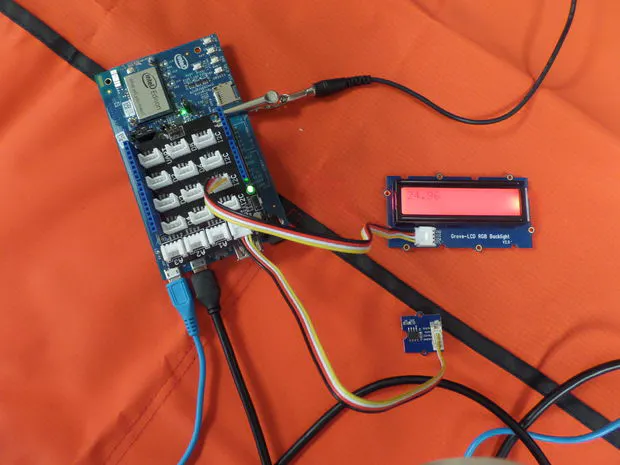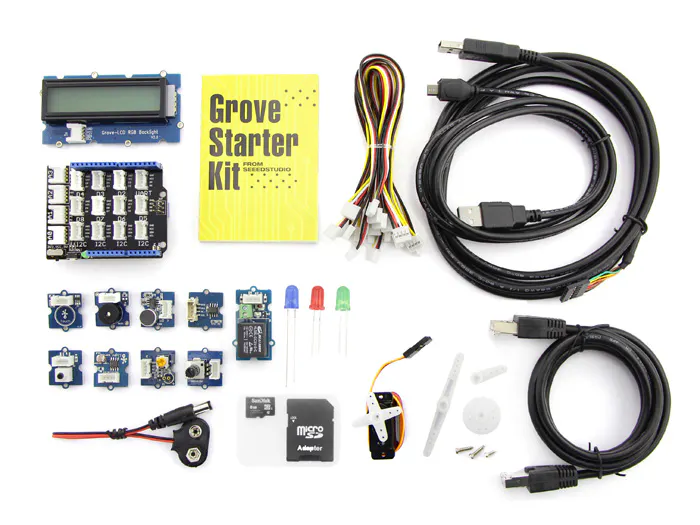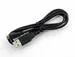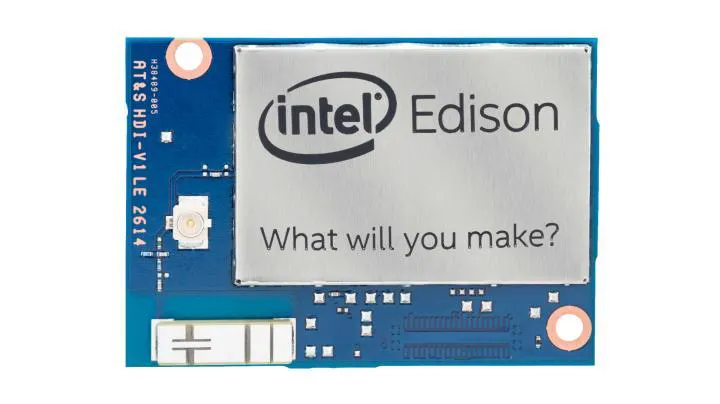Published

# COI - Temperature Monitor

You will need an Intel Edison for Arduino, a Grove Starter Kit Plus and a computer with the Edison-Arduino IDE installed.

Full instructions provided422## Things used in this project

### Hardware componentsArduino UNO & Genuino UNO
×1Seeed Grove starter kit plus for Intel Edison
×1USB-A to Micro-USB Cable
×1Intel Edison
×1

## Code

### Temperature_Mood_Lighting.ino

Arduino
```#include <Wire.h>
#include <rgb_lcd.h>
#include <math.h>
rgb_lcd lcd;

int tempSense = 0;
int B=3975; //This is a constant used to convert between the thermistor resistance in the temperature sensor and the actual temperature in Celcius.
double minTemp = 10;
double ideal = 20;
double maxTemp = 25;

void setup() {
lcd.begin(16, 2);
lcd.setRGB(255, 255, 255);
pinMode(tempSense, INPUT);
}

void loop() {
lcd.setCursor(0,0);
lcd.print("                                ");//32 spaces clears screen of all text - deals with text offset glitches
lcd.setCursor(0,0);
double temperature=1/(log(resistance/10000)/B+1/298.15)-273.15;
lcd.print(temperature);

temperature = min(temperature, maxTemp);
temperature = max(temperature, minTemp);
double fraction;
if(temperature < ideal){
fraction = (ideal - temperature) / (ideal - minTemp) * .5 + .5;
} else {
fraction = (maxTemp - temperature)/(maxTemp-ideal) * .5;
}
int r = 0;
int g = 0;
int b = 0;
double quarter = 1.0/4.0;
if(fraction < quarter){
r = 255;
g = (int)((fraction)/quarter * 255);
} else if(fraction < 2 * quarter){
r = (int)((2 * quarter - fraction)/quarter * 255);
g = 255;
} else if(fraction < 3 * quarter){
g = 255;
b = (int)((fraction-2*quarter)/quarter * 255);
} else{
g = (int)((4 * quarter - fraction)/quarter * 255);
b = 255;
}

//Set LCD Colors
lcd.setRGB(r,g,b);

delay(500);//Wait half a second.
}
```